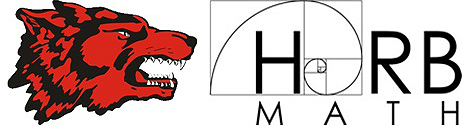# RC2: A.3A Slope from a table

10 Questions
RC2: A.3A

Determine the slope of a line given a table of values, a graph, two points on the line, and an equation written in various forms, including y = mx + b, Ax + By = C, and y – y1 = m(x – x1)

Student ID